# Calculating Velocity in the Toolbox

Member, Administrator, Moderator Posts: 63 admin
edited July 2020

This example uses the train data (https://community.endaq.com/discussion/75/vibration-from-bridge-as-train-passes-overhead#latest) to calculate velocity in three ways:

• Straight integration and double integration
• Calculating a PSD, then integrating that
• Calculating a shock response spectrum pseudo velocity which tries to define severity (admittedly this is better for shock, not vibration data)

Here is the video of the analysis:
https://info.endaq.com/hubfs/velocity-integration.mp4

## Double Integration

First is the x axis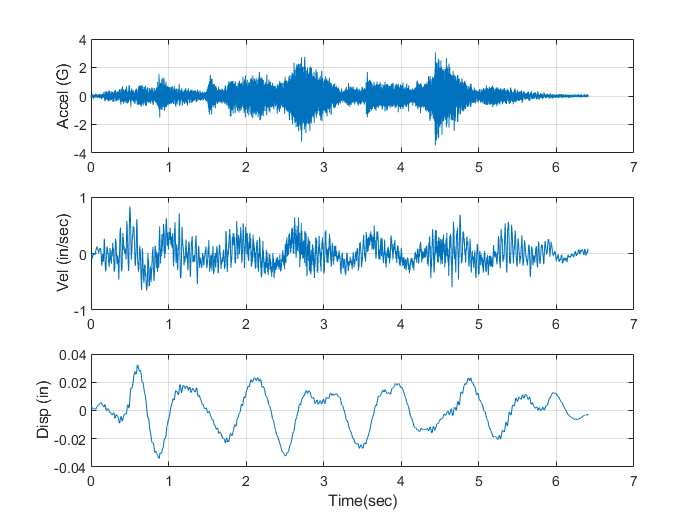Then the y axis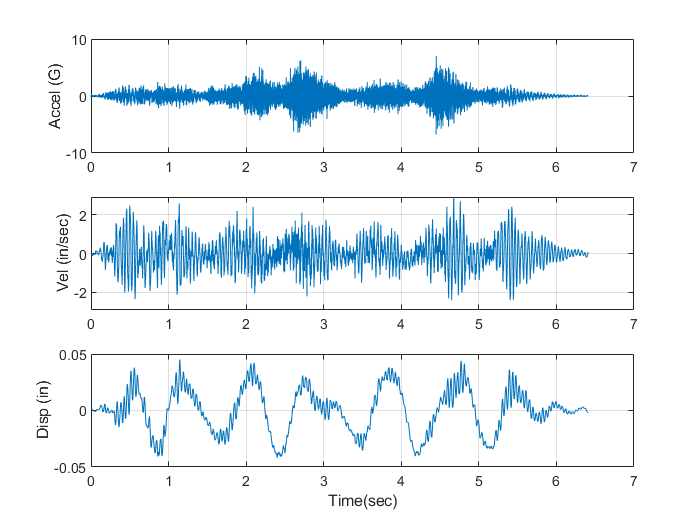Now the z axis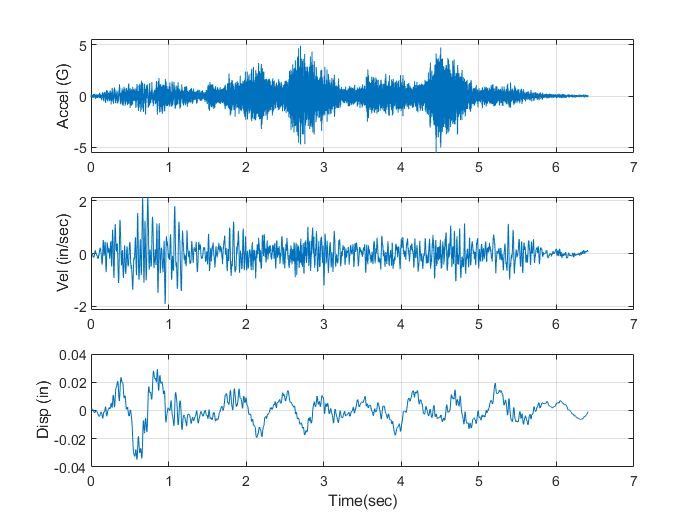## PSD

The RMS of each is calculated:

• X Axis: 0.4g
• Y Axis: 1.0g
• Z Axis: 0.7g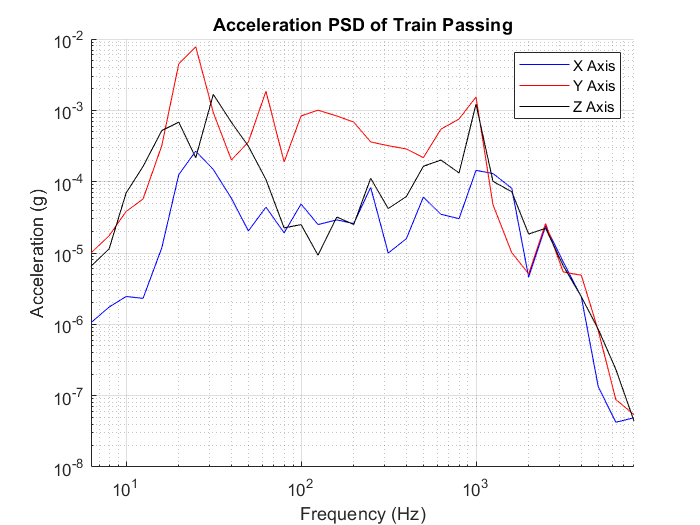Now calculating the PSD in velocity, here is the RMS:

• X Axis: 0.15 in/s
• Y Axis: 0.70 in/s
• Z Axis: 0.38 in/s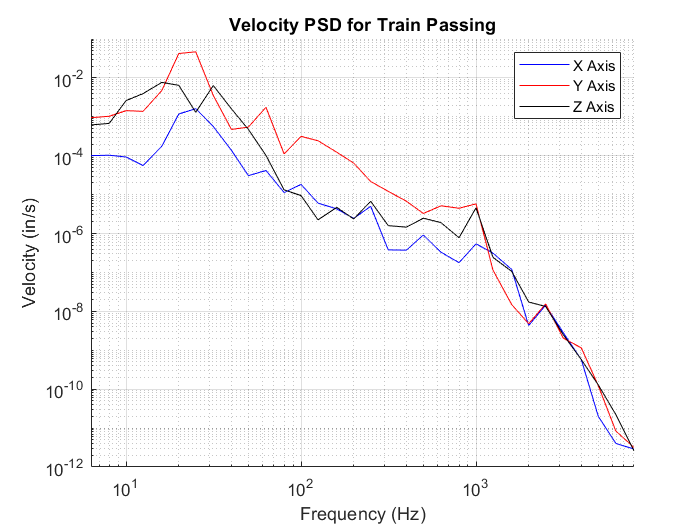## Pseudo Velocity Shock Response Spectrum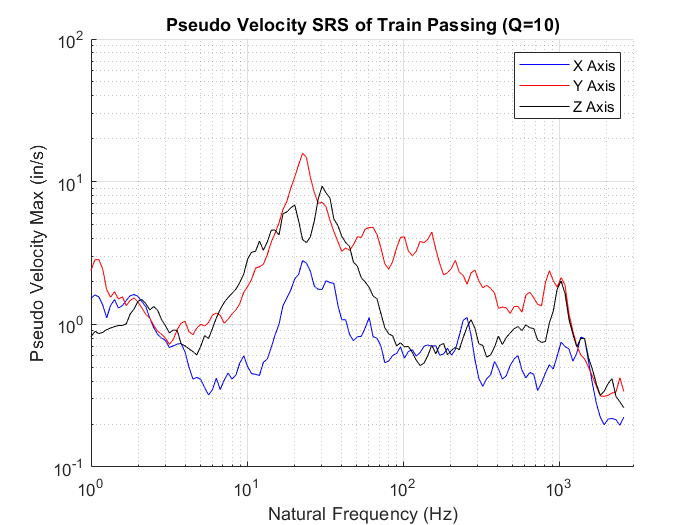Tagged:
Sign In or Register to comment.